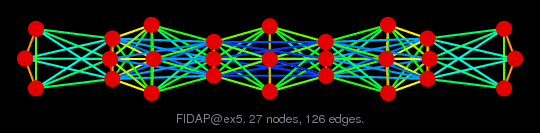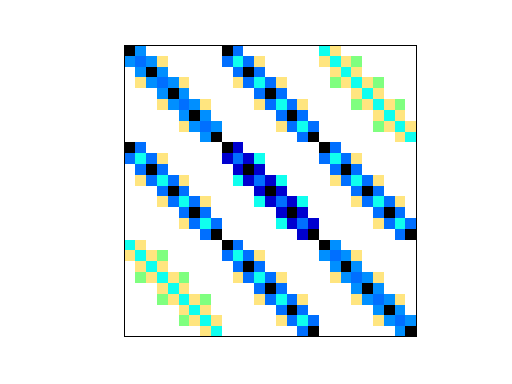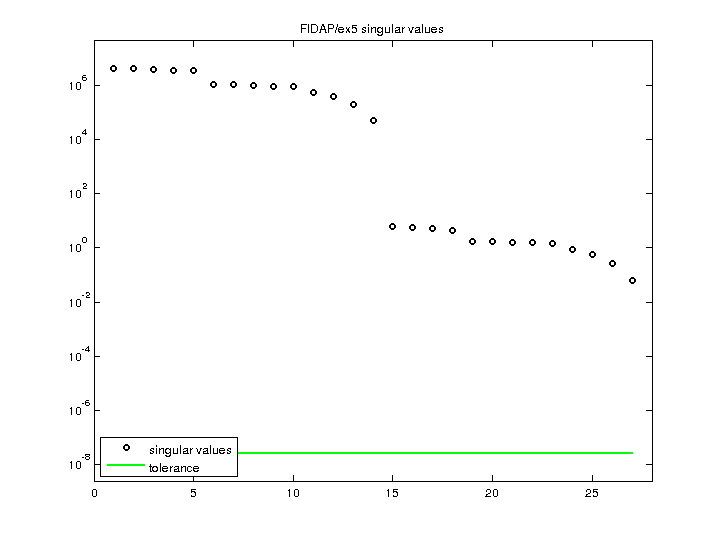Matrix: FIDAP/ex5

Description: TEST MATRIX FROM FIDAP: EX5.MAT(undirected graph drawing)• Matrix group: FIDAP
• download as a MATLAB mat-file, file size: 1 KB. Use UFget(436) or UFget('FIDAP/ex5') in MATLAB.

 Matrix properties number of rows 27 number of columns 27 nonzeros 279 structural full rank? yes structural rank 27 # of blocks from dmperm 1 # strongly connected comp. 1 explicit zero entries 0 nonzero pattern symmetry symmetric numeric value symmetry symmetric type real structure symmetric Cholesky candidate? yes positive definite? yes

 author A. Baggag, Y. Saad editor A. Baggag, Y. Saad date 1994 kind computational fluid dynamics problem 2D/3D problem? yes

 Ordering statistics: result nnz(chol(P*(A+A'+s*I)*P')) with AMD 153 Cholesky flop count 9.8e+02 nnz(L+U), no partial pivoting, with AMD 279 nnz(V) for QR, upper bound nnz(L) for LU, with COLAMD 153 nnz(R) for QR, upper bound nnz(U) for LU, with COLAMD 261

 SVD-based statistics: norm(A) 4.35957e+06 min(svd(A)) 0.0655578 cond(A) 6.64996e+07 rank(A) 27 sprank(A)-rank(A) 0 null space dimension 0 full numerical rank? yes

 singular values (MAT file): click here SVD method used: s = svd (full (A)) status: ok